# Exploring H-1B Data with R: Part 2

Learn even more about exploratory data analysis with R in the second part of the "Exploring H-1B Data" tutorial series
Jan 2017  · 14 min read

DataCamp's blog "Can Data Help your H-1B Visa Application" presented you with some of the results of an analysis of H-1B data over the years. Now, it's time to get started yourself! Ted Kwartler will guide you through this with a series of R tutorials.

Picking up at our last post I want to show you how to add more visuals to your Exploratory Data Analysis (EDA) work and introduce some new functions. Last time you learned how to web scrape 1.8m H1B visa applications, correct the variable types, examine some rows and create basic visualizations like barplot and scatterplot.

For this post I will assume you were able to load the data using `fread`, correct the variables to be dates, change salary to numeric and create new data features like `year` and `month`.

First, add some visualizations libraries.

``````library(ggplot2)
library(ggthemes)``````

## Numeric Exploratory Data Analysis: Base Salary

The summary function is a good catch-all when doing EDA work. In this case, you apply the `summary()` function to a numeric vector `\$base_salary`. In addition to numeric values, you can apply the `summary()` function to strings and factors to get appropriate descriptive stats. You can even apply it to entire data frames to get column-wise statistics. Here `summary()` will print the minimum, 1st and third quartile, median, mean and maximum values for H1B salaries.

``summary(h1b.data\$base_salary)``

In the summary printout, you should note the maximum value is 7,278,872,788. This definitely seems like a mistake! One way to find the row with the maximum value is with `which.max()`. The `which.max()` function determines the row with the first maximum value within the vector it is given, `\$base_salary`.

In the code below, you use this row number to index the data frame so the entire record is printed.

``h1b.data[which.max(h1b.data\$base_salary)]``

That is one well paid IBM Software Engineer. Not surprisingly, the H1B case status is “withdrawn”. A quick way to remove an outlier like this is simply to pass in a negative sign along with the `which.max` statement. Just for educational purposes, you can verify the number of rows in the data frame with `nrow` before and after the maximum salary is removed.

``````nrow(h1b.data)
h1b.data<-h1b.data[-which.max(h1b.data\$base_salary)]
nrow(h1b.data)``````

Removing a single row is tedious and often is not the best way to remove outliers. Instead, you can plot the salaries to understand the data distribution visually. In this code the innermost function is sort. `Sort()` accepts the salary vector and by default, `sort()` will arrange values in ascending order. The next innermost function is the `tail()` function. In this example, `tail()` will take the sorted vector and subset it to the last 50 values. Since the order is ascending, `tail()` will sort the 50 largest salaries. Lastly, base R’s plot function will automatically create a dot plot of the 50 salaries.

``plot(tail(sort(h1b.data\$base_salary),50))``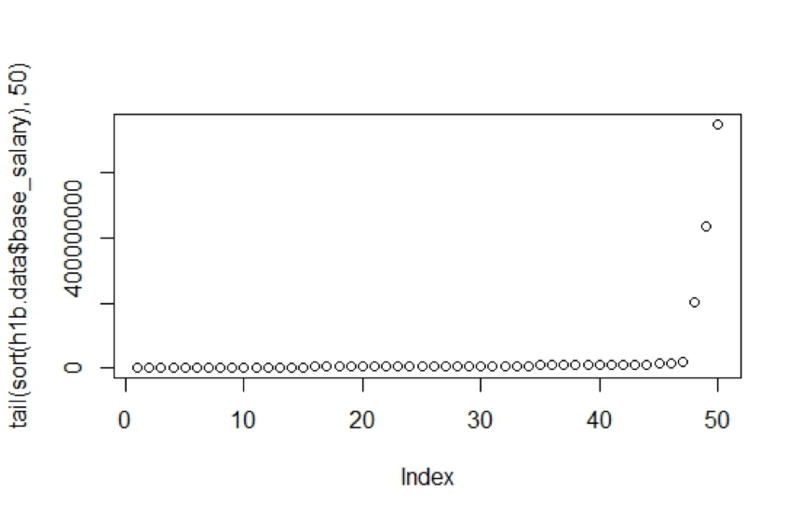Even with the maximum salary removed, the last 5 salaries range from \$10m to \$750m.

In this next section, you can learn how to create an arbitrary cutoff using an `ifelse` statement. This is an efficient way to remove outliers instead of one at a time.

An `ifelse` statement works like this: if some logical declaration is `TRUE` then perform an action, otherwise (else) perform another action.

In this code you are rewriting the `\$basesalary` vector using `ifelse`. Then you call `summary()` on the vector to display how the `ifesle` statement changed the output.

Specifically, the code follows this logic: if a `\$base_salary` is greater than `\$250,000` then change it to `NA`, otherwise use the original `\$base_salary`.

``````h1b.data\$base_salary<-ifelse(h1b.data\$base_salary>250000,NA,h1b.data\$base_salary)
summary(h1b.data\$base_salary)``````

Notice the summary output now adds 7,137 NA’s to the other descriptive statistics. Furthermore, the maximum value is now 250000.

For continuous data, you can explore the distribution with a kernel density plot. A kernel density plot estimates the population distribution from a sample. Here you are first using `ggplot` to create a base visual layer.

Start off by passing in the `h1b.data` and then set the `X` aesthetic with the column name `base_salary`. Next, add a `geom_density` layer to create the kernel density plot. Within the parameters, the code below specifies a fill color, `“``darkred``”` and `'NA'` for the outline color. In the last layers, you employ ggtheme’s gdocs theme and remove the Y axis text.

``````ggplot(h1b.data,aes(base_salary)) +
geom_density(fill='darkred', color='NA') +
theme_gdocs() +
theme(axis.text.y=element_blank())``````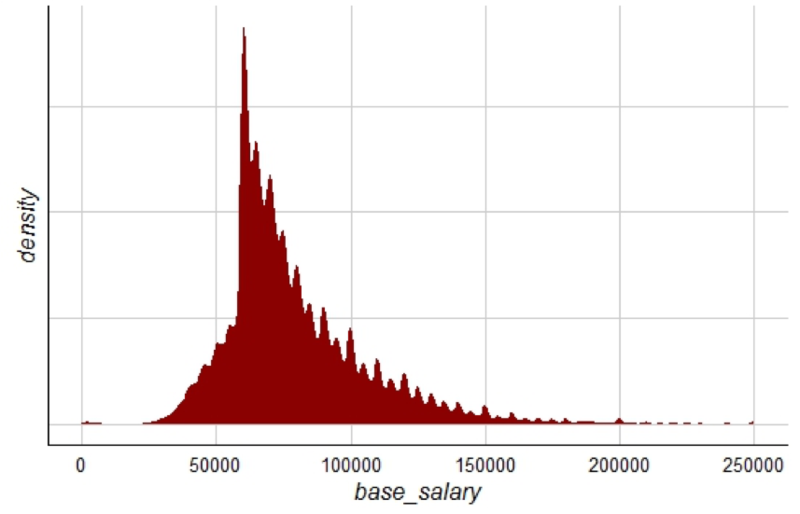You see that the H1B Salaries are mostly above \$50K but below \$100K.

## Categorical EDA: Case Status

Now that you have a basic understanding to explore numeric values you can transition to categorical values.

A categorical value represents a unique class attribute. For example, a day of the week could be Monday, Tuesday, Wednesday, etc. from the 7 daily “levels”. In R, categorical values can mistakenly become strings which means they are all unique even if you are talking about multiple days that are “Tuesday”. In contrast, two categorical Thursdays share the same attribute in that they are both share the “Thursday” level.

In this section, you will review the `\$case_status` vector. H1B visa data repeats in this column leading one to believe they are factors of the application status instead of unique strings. To examine this information, let’s ensure the vector is a factor using `as.factor()`. This changes the class data to a categorical value. Next, you use `table()` to tally the information:

``````h1b.data\$case_status<-as.factor(h1b.data\$case_status)
table(h1b.data\$case_status)``````

Once again, values are not evenly distributed. 1.7m of the 1.8m rows are “CERTIFIED.” Another 95k are “WITHDRAWN” or “DENIED” while the remaining factor levels total less than 20.

The code below demonstrates how to remove the outlier categorical values. First, you create a small vector, drops, containing the factor levels to remove. Each of the factor levels is separated by a pipe “|” operator. The pipe is a straight line above your enter key. In logical expressions, the symbol represents “OR.”

So, the `drops` object is saying “INVALIDATED OR PENDING QUALITY… OR UNASSIGNED OR REJECTED”.

``drops<-c('INVALIDATED|PENDING QUALITY AND COMPLIANCE REVIEW - UNASSIGNED|REJECTED')``

Next, you use `grepl()` to declare a `TRUE` if one of the rows contain a level from `drops`. The `grepl()` command is an old UNIX command that searches for a pattern. The output is a `TRUE` if the pattern is found and `FALSE` if it is not.

In this case, you are passing in the text from drops as search patterns. In the next parameter, you apply `grepl()` to the `\$case_status` vector.

Note that the exclamation mark to begin the `grepl` expression: this symbol represents a negation.

Putting it all together, the negation occurs when `grepl` returns a `TRUE`:

``h1b.data<-h1b.data[!grepl(drops,h1b.data\$case_status),]``

R retains unused levels even if they are removed. In this case, calling table on the `h1b.data\$case_status` will still show “REJECTED” with a 0. One method to drop unused levels is to call factor on the vector.

You can re-check the tally by using `table()` again:

``````table(h1b.data\$case_status)
h1b.data\$case_status<-factor(h1b.data\$case_status)
table(h1b.data\$case_status)``````

One way to visualize the new factor distribution is with the data visualization library `ggplot`.

In the first layer, you pass in the data frame and set aesthetics using `aes`. This creates the base `status.plot` for the visual. The next layer adds a geometric bar shape with `geom_bar`, then flips the x,y axis with `coord_flip` and finally adds thematic information with `theme_gdocs()`. The GDocs theme is a shortcut for a predefined color palette. In the last layer, you adjust the GDocs theme by removing the Y Axis title and removing the legend.

``````status.plot <- ggplot(h1b.data, aes(factor(case_status),fill=factor(case_status)))
status.plot +
geom_bar()+
coord_flip()+
theme_gdocs()+
theme(axis.title.y=element_blank(),legend.position="none")``````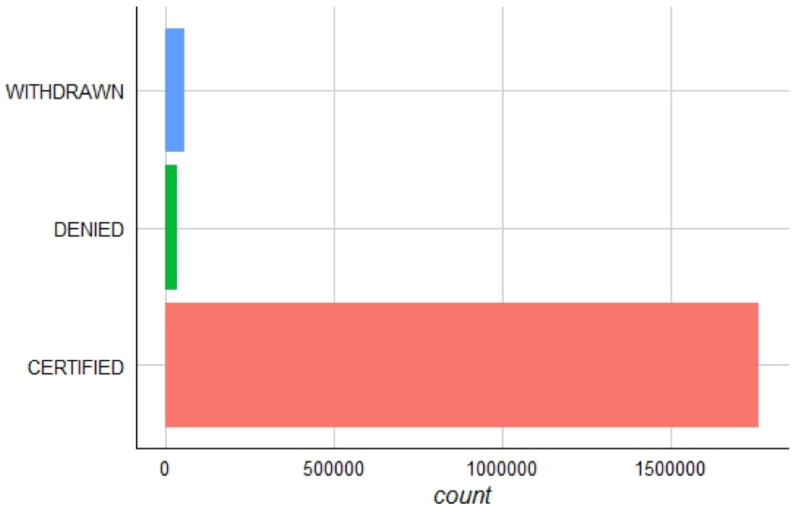This plot compares the H1B case status factors.

### Salary & Status: Numeric by Factor EDA

Another way you can explore data is by looking at the variable interactions. This is particularly helpful if you are doing predictive modeling and can examine inputs by the dependent variable.

In this section, you will explore salary distributions by H1B case status.

In this code, you are building a boxplot. A box plot is a compact way to review population distributions.

The figure below places a normal distribution on the left vertically with a boxplot on the right. In a box plot the population median is represented as a dark line inside the box. The line segments the population into 2nd and 3rd quartiles. The “whiskers” extending outside the box represent the 1st and 4th quartiles. Lastly, the dots are meant to show true outliers to the distribution.This is a normal distribution alongside a box plot for comparison.

You can construct a boxplot with ggplot and passing in the H1B data. The `geom_boxplot()` function is the layer that creates the box plot distributions. Within this function, you set the aesthetics of the X axis as case_status with values such as “CERTIFIED”. Within `aes`, the Y axis is the `base_salary` and the last parameter simply color codes the different factors.

The next section of code illustrates a third method for outlier removal. Previously, you used `which.max()` and `ifelse` to remove outliers.

In a ggplot visual, you can also omit records by limiting the axis.

Here, you are using `ylim` and passing in `0,100000`. This means the Y axis will only extend to \$100,000 and ggplot will ignore any others. Lastly, as before, you add theme_gdocs coloring and adjust the theme by removing the X axis text.

``````ggplot(h1b.data) +
geom_boxplot(aes(factor(case_status), base_salary, fill=as.factor(case_status))) +
ylim(0,100000) +
theme_gdocs() +
scale_fill_gdocs() +
theme(axis.text.x=element_blank())``````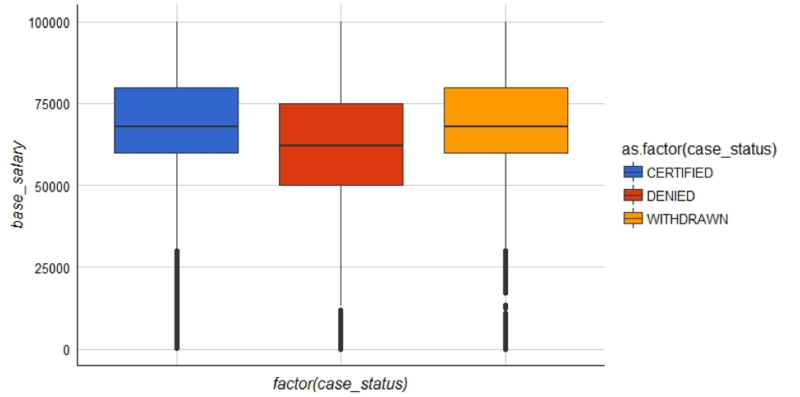The boxplots show DENIED H1B applications have a lower salary. It also appears that salaries above \$75,000 have a better chance of being CERTIFIED.

## Temporal EDA: Filing Time

Still another way to explore this data is by time. Assuming you want to know when to apply for your H1B visa you will need to focus on the CERTIFIED applications. Using subset, pass in the entire data frame and then a logical condition.

Any rows where the case_status is equal to “CERTIFIED” will be retained in `case.month`.

``case.month<-subset(h1b.data,h1b.data\$case_status=='CERTIFIED')``

Now you will need to describe the certified applications along two dimensions, month and year.

You will use `tapply()` to pass in `case.month\$case\$status` and then put the other two dimensions into a list. The last parameter is the function to apply over the array. In this case, `length` will return the number of CERTIFIED H1B applications by year by month. In the second line you call `case.month` to display the values.

``````case.month<-tapply(case.month\$case_status, list(case.month\$submit_month,case.month\$submit_yr), length)
case.month``````
2012 2013 2014 2015 2016
Apr NA 29021 29687 32498 32961
Aug 5 22468 25903 27916 31290
Dec 14635 18188 22676 24990 NA
Feb NA 35837 52032 70787 77198
Jan NA 22021 25268 29550 30090
Jul 2 23662 27396 31604 26521
Jun NA 22356 27529 33120 33565
Mar NA 98456 112511 139680 162984
May NA 24688 27115 29131 30854
Nov 16240 20727 20235 19597 NA
Oct 18493 16714 24529 23156 NA
Sep 3471 20342 25913 25825 20240

It is easier to plot this data if you reshape it with data.table’s `melt()` function. This code will put the month’s years and values into three columns. You can examine the new shape with `head()`.

``````case.month<-melt(case.month)

Optionally you can change the months to numeric values using the constant `month.abb` within `match()`. Here, you rewrite `Var1` from month abbreviations to numbers. If you do this, you lose the month labels in the plot but the lines are in temporal order not alphabetical by month name:

``case.month\$Var1<-match(case.month\$Var1,month.abb)``

Next create a timeline for the temporal exploration.

First, pass in the `case.month` object. Next, you add the X axis representing month, then group and color by year. Then add in ggtheme aesthetics like before. The theme is adjusted to remove the x title and finally the last layer specifies the X axis should have integers 1 to 12 representing months.

``````ggplot(case.month) +
geom_line(aes(Var1,value,group=as.factor(Var2),color=as.factor(Var2))) +
theme_gdocs() +
scale_color_gdocs() +
theme(axis.title.x=element_blank())+
scale_x_continuous(breaks=seq(1,12))``````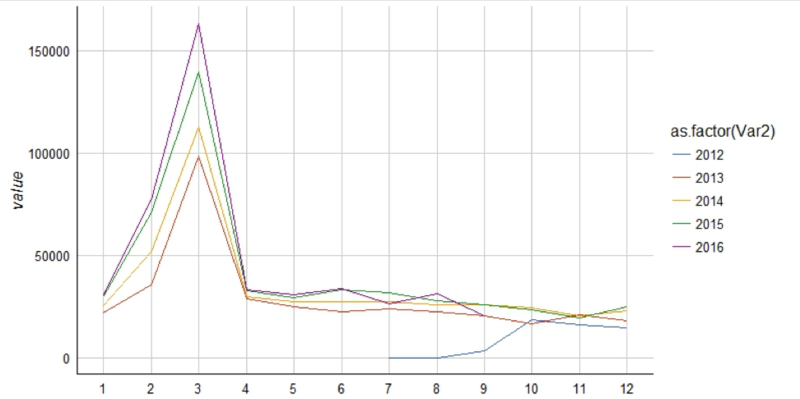March (3rd month) stands out as a peak for getting H1B applications certified.

Now that you know your application has a good chance of being CERTIFIED in March you need to know how long it takes to process.

That means you need to calculate the difference between `submit_date` and `start_date`. You can use `difftime()` to calculate the differences between date vectors. First, you pass in the `submit_date` then the `start_date`. The last parameter declares the time unit.

``submit.start<- difftime(h1b.data\$submit_date ,h1b.data\$start_date , units = c("days"))``

Once you have the time difference for each H1B application you can print the mean and median. According to this data, you should submit your application about 75-90 days before March to improve your chances!

``````mean(submit.start)
median(submit.start)``````

## Bonus: Working on more than One Feature

The last way I will demonstrate working with this data is to examine the top employers, job titles and locations so you can focus your employment efforts there.

To quickly gather frequency values for multiple features, you can write a function instead of redundant code.

For this analysis you create a vector of column names.

``h1b.features <- c('employer','job_title','location')``

Next, you write a function called `freq.get`. Passing in the vector name as `x`, `freq.get` constructs a tally for that column using `table()`. Next, the vector is sorted, names are applied and data frame is constructed. Lastly, the data frame is reorder from top to bottom.

``````freq.get<-function(x){
f.table<-table(h1b.data[[x]])
f.table<-sort(f.table, decreasing=TRUE)
f.names<-names(f.table)
f.df<-data.frame(names=f.names,freq=f.table)
f.df<-f.df[order(f.df\$freq,decreasing = T),]
return(f.df)
}``````

Rather than apply this function to each column, you use `pblapply()` from the `pbapply` package. Pass in `h1b.feature`, and then `freq.get`. The output is a list of 3 frequency data frames. You add list names using names in the second line.

``````all.freq <- pblapply(h1b.features, freq.get)
names(all.freq)<-h1b.features``````

Now you can easily review the most frequent values from each of the columns. Remember you could have done this on each column individually but in wide data sets writing a function saves time and is less error prone.

``````head(all.freq\$employer,12)

## Conclusion

I hope you found these EDA posts interesting. Along the way, you learned how to web scrape data, correct the variable classes and finally basic functions to get descriptive stats. As a visual learner, I always look for interesting and insightful visualizations when doing EDA so hopefully these posts added to your visualization skillset.

If you are inclined you could create a cool H1B visualization with this data and tweet it to me @tkwartler!

Stay tuned for another part in the "Exploring H-1B Data with R" tutorial series! In the meantime, consider checking out our data.table cheat sheet, our R data frame tutorial, our Data Analysis in R, the data.table Way course or our Data Manipulation in R with dplyr course.

### Introduction to R

Beginner
4 hours
2,352,315
Master the basics of data analysis in R, including vectors, lists, and data frames, and practice R with real data sets.
See Details

### Intermediate R

Beginner
6 hours
521,779
Continue your journey to becoming an R ninja by learning about conditional statements, loops, and vector functions.

### Exploratory Data Analysis in R

Beginner
4 hours
78,701
Learn how to use graphical and numerical techniques to begin uncovering the structure of your data.
See More